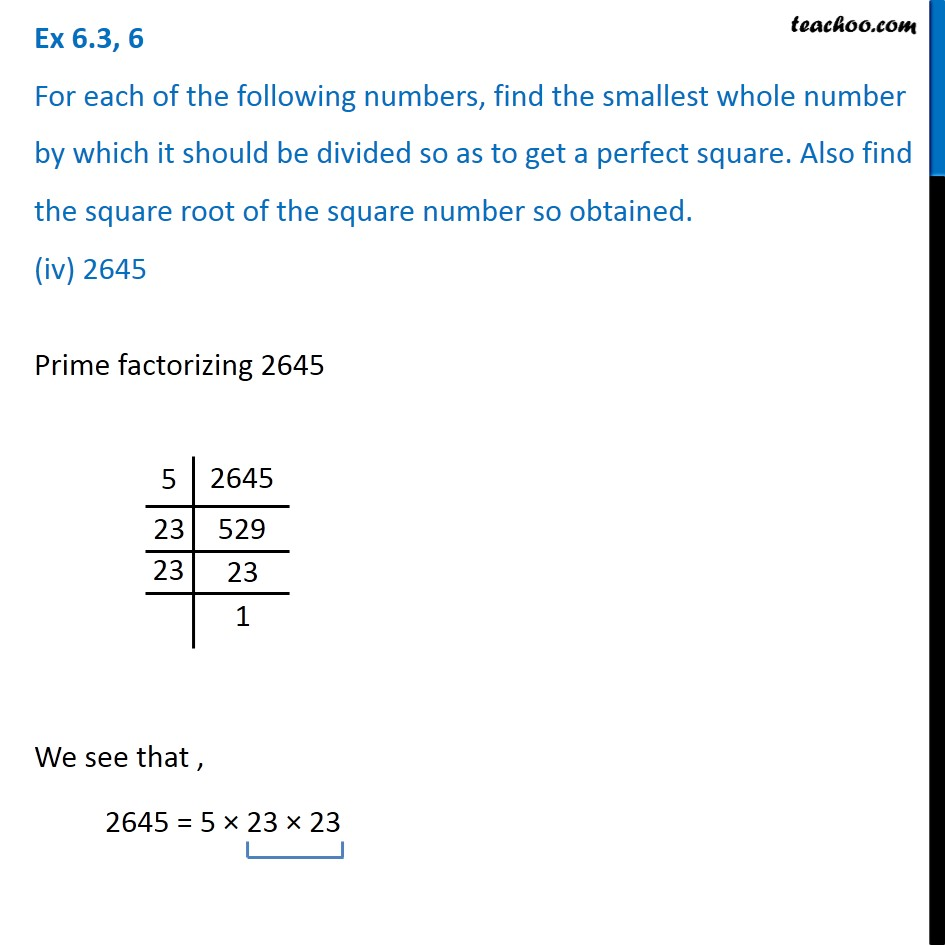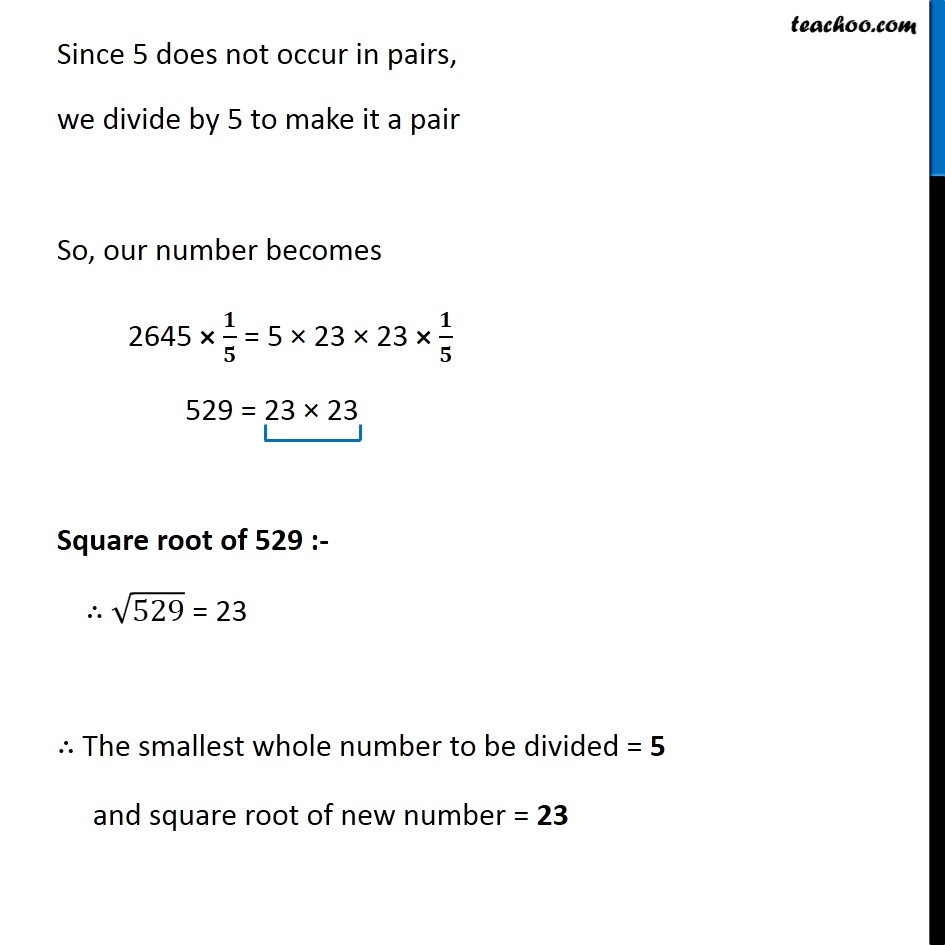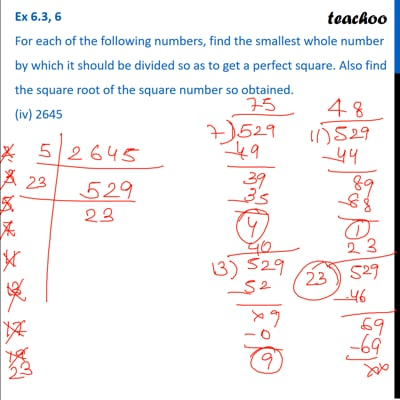Ex 6.3

Chapter 6 Class 8 Squares and Square Roots
Serial order wiseThis video is only available for Teachoo black users

### Transcript

Ex 6.3, 6 For each of the following numbers, find the smallest whole number by which it should be divided so as to get a perfect square. Also find the square root of the square number so obtained. (iv) 2645Prime factorizing 2645 We see that , 2645 = 5 × 23 × 23 Since 5 does not occur in pairs, we divide by 5 to make it a pair So, our number becomes 2645 × 𝟏/𝟓 = 5 × 23 × 23 × 𝟏/𝟓 529 = 23 × 23 Square root of 529 :- ∴ √529 = 23 ∴ The smallest whole number to be divided = 5 and square root of new number = 23